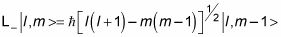##### Quantum Physics For Dummies, Revised EditionIn quantum physics, you can find the eigenvalues of the raising and lowering angular momentum operators, which raise and lower a state’s z component of angular momentum.

Start by taking a look at L+, and plan to solve for c:

L+| l, m > = c | l, m + 1 >

So L+ | l, m > gives you a new state, and multiplying that new state by its transpose should give you c2: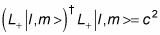To see this equation, note that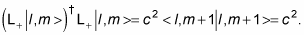On the other hand, also note that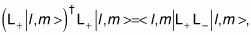so you have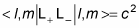What do you do about L+ L? Well, you assume that the following is true: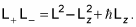So your equation becomes the following: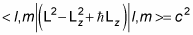Great! That means that c is equal to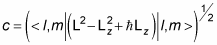So what is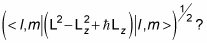Applying the L2 and Lz operators gives you this value for c: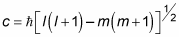And that’s the eigenvalue of L+, which means you have this relation: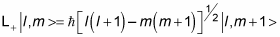Similarly, you can show that L gives you the following: